# GetFem++

## GetFem++

GetFEM++ is an open source library based on collaborative development. It aims to offer a framework for solving potentially coupled systems of linear and nonlinear partial differential equations with the finite element method.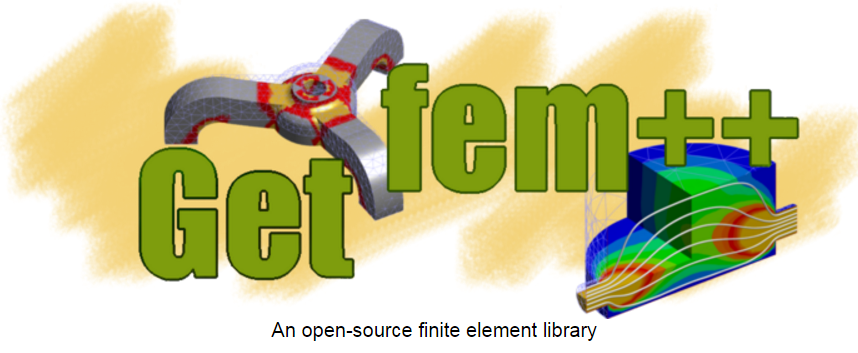SuperLU is a general purpose library for the direct solution of large, sparse, nonsymmetric systems of linear equations on high performance machines.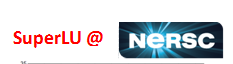## Finite Element Method-Mild Slope Equation (FemMse)

• A generic FEM code (Tsai and Chou, 2015) is implemented for solving the MSE-type models of FemMse. Furthermore, quartic elements are adopted in the FEM numerical solution procedures.

• The present finite element model is generic. To be more clear, template parameters are implemented in the present model, such as the mild-slope equation type, bathymetry, dimensionality and others.
• We compare our result (CCMS) with the EMM (Tsai et al., 2011) and the IEM (Porter and Porter, 2000).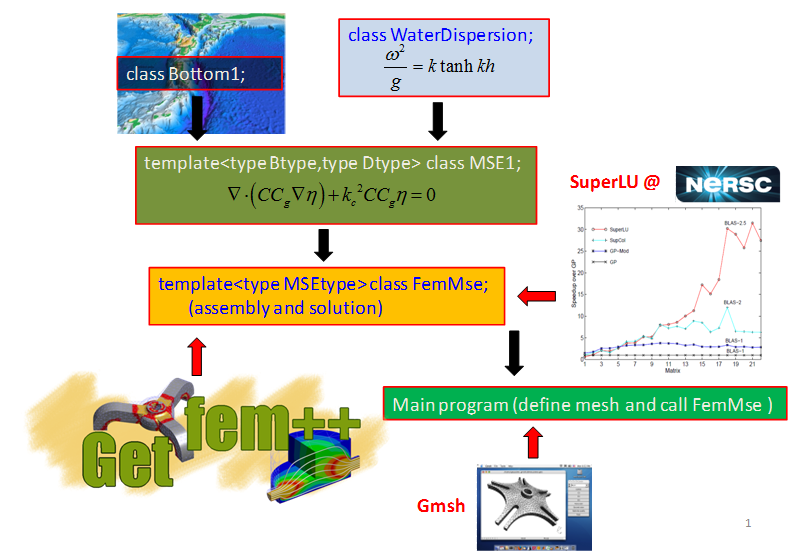### Numerical Results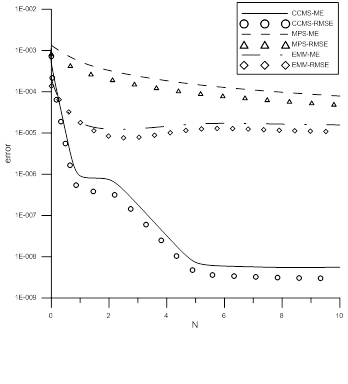IEM solutions for the short-wave sinusoidal slope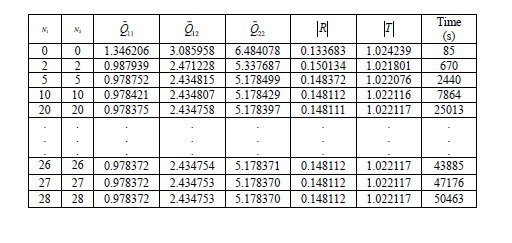Reflection coefficients for the sinusoidal shoal### Reference

Chia-Cheng Tsai, and Wan-Rong Chou (2015) . Comparison between consistent coupled-mode system and eigenfunction matching method for solving water wave scattering. Journal of Marine Science and Technology, 23 , 870-881 Tsai, C.-C., Hsu, T.-W. & Lin, Y.-T. (2011) On step approximation for Roseau’s analytical solution of water waves. Mathematical Problems in Engineering. Porter, R. & Porter, D. (2000) Water wave scattering by a step of arbitrary profile. Journal of Fluid Mechanics, 411, 131-164.

Back